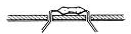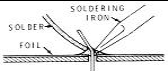EXPT NO:- 3

Title:- Build and test amplifier designed in Expt. No. 2

Objective â€“ To build and test the single stage CE amplifier designed in expt. no. 2 and measurement

of different parameters such as gain, input impedance, output impedance.

Pre-requisites:- Basics of PCB design

Biasing of BJT

Instruments & Components:- Resistors , Capacitors ( 10uF), Transistor (BC 547),

Theory:-

PCB Design -

1. Component mounting. - Components are pushed through from the top side of the board and the leads are bent slightly to hold the component while soldering.1. Components are then soldered to the board as shown in Figures.

Steps of soldering -

a) The soldering iron tip should be placed in contact with both the trace (foil) and the lead. The

two should be heated only enough to melt solder in order to avoid damaging sensitive

components and to avoid delamination of the PCB traces.

b) Solder is then touched to the area and allowed to flow freely around the lead and to cover the

solder pad. A minimal amount of solder should be applied. Only enough solder to cover the

joint and to form a smooth fillet should be used.

c) The iron should be removed after the solder has flowed properly and wetted all surfaces. The

component and the board should not be moved until the solder has hardened (up to several

seconds, depending on the lead and trace size).Procedure:-

I] DC Analysis :

1) Build the circuit as shown in fig. a) Apply Vcc=10Volt and measure V CEQ , VBEQ, ICQ with multimeter

2) Compare the values with designed values.

II] AC Analysis :

1) Voltage Gain (Av)-

Apply input signal Vs=50mVp-p from function generator and measure Vo on CRO. Calculate voltage gain Av=Vo/Vs

2) Current Gain (Ai)-

Connect 1k resistor in series with input signal Vs and measure voltage across resistor Vb and output Voâ€™ on CRO. Calculate

Ii= (Vs-Vb)/1K Io= Voâ€™/Rc

Ai= Io/Ii

3) Input Impedance (Ri)

Calculate Ri=Voâ€™/(Vo-Voâ€™) X 1k.

4) Output Impedance (Ro)

Disconnect 1K resistor and Connect 10k resistor across the output and measure output Voâ€™â€™. Calculate Ro=[(Vo-Voâ€™â€™)/Voâ€™â€™] X 10k.

5) Calculate Vo/âˆš2 . Measure lower cut of frequency(FL) and upper cut off frequency(FH) at which output falls to Vo/âˆš2. Calculate

Bandwidth = FH - FL

Observations:- Vs=30mVp-p

 Sr. No. Quntity Measured values 1 Vo â€¦â€¦ V 2 Vb â€¦â€¦.V 3 Voâ€™ â€¦â€¦ V 4 Voâ€™â€™ â€¦â€¦.mV 5 FL â€¦ Hz 6 FH â€¦â€¦..Hz

Calculations :

1) Av= Vo/Vs

2) Ri=Voâ€™/(Vo-Voâ€™) X 1k.

3) Ro=(Vo-Voâ€™â€™)/Voâ€™â€™ X 10k.

4) Bandwidth = FH-FL

Result:-

I] DC Analysis -

 Sr. No. Quantity Values Practical Designed(expt no.2) 1 ICQ 2 VCEQ 3 VBEQ

II] AC Analysis -

 Sr. No. Parameter Values 1 Voltage gain (Av) 2 Input Impedance (Ri) 3 Output Impedance (Ro) 4 Bandwidth (B.W.)